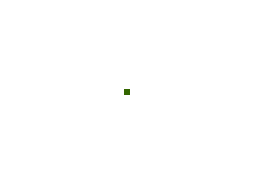# Midgard, Grass Growth, Unit Tests

Now that I can display the growing grass, something a little less exciting than watching grass grow: taking a step back and adding unit tests. As much as I like the data structure for storing the growth of the grass field, it is difficult to reason about. Therefore, unit tests.

Clearly, the best way to avoid bugs in code is by adding more code, so I’m adding a new utility function num_filled(). This counts the total number of tiles filled anywhere on the field. This will be useful later on for determining if any large-scale deforestation is ongoing. For now, it is useful for determining if the growth is working correctly.

A single tile will grow to cover the adjacent tiles, leading to 5 total tiles filled. The next iteration, it will cover 13, then 25, as it grows outward. This follows the formula $a(n) = 2n(n+1) + 1$. This can be derived by noticing that there are four triangles, in a separate quadrant, of size $n$, along with the central tile. The triangular numbers are $\frac{n(n+1)}{2}$. Adding together all four triangles, plus the one tile in the center, gives the final formula.

Or, you can test the first few iterations, plug it into OEIS, and find that these are known as the “centered square numbers”.There is now a unit test to ensure that the growth follows this pattern, along with a few others to make sure that it continues working at tile boundaries.